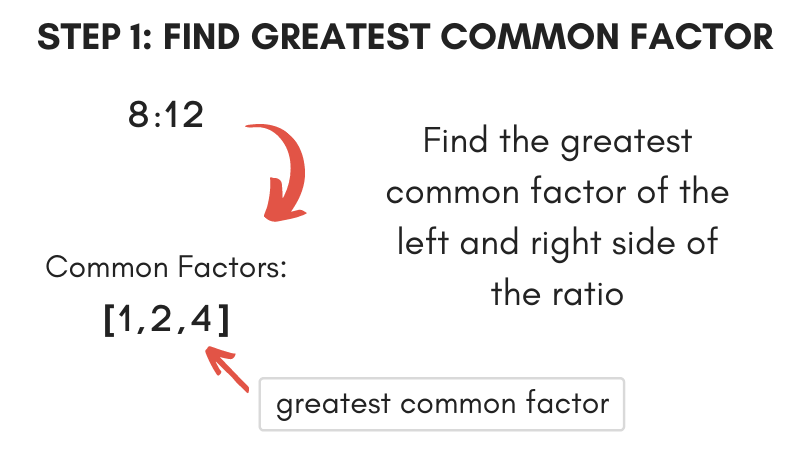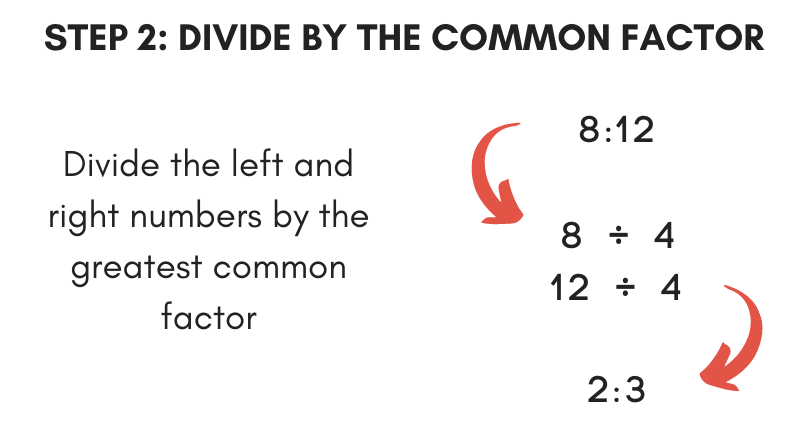# Ratio Simplifier

Simplify a ratio to reduced form by entering it in the ratio simplifier calculator below.

Ratio
:

## How to Simplify a Ratio

Like fractions, ratios sometimes need to be simplified to a reduced form. The calculator above easily reduces a ratio, but you can do it yourself in two easy steps.

These steps are the same as the steps to simplify a fraction.

### Step One: Find the Greatest Common Factor

The first step to simplify a ratio is to find the greatest common factor. There are two things you need to do to find the greatest common factor.

First, find all of the numbers that divide evenly into the ratio’s left and right sides; these are the common factors. Then, choose the largest common factor from the set; this is the greatest common factor.You can also use our greatest common factor calculator.

### Step Two: Divide Left and Right by the Common Factor

The second step to simplify a ratio is to divide the ratio’s left and right sides by the greatest common factor. This will reduce the ratio to the smallest possible form, keeping both parts of the ratio as whole numbers.For example, let’s simplify the ratio 6:8.

Start by finding the greatest common factor.

Factors of 6: [1,2,3,6]
Factors of 8: [1,2,4,8]

The biggest number in each set is 2.

Divide each side of the ratio by the common factor.

6 ÷ 2 = 3
8 ÷ 2 = 4

Thus, 3:4 is the simplified form.

You might also find our ratio calculator useful for solving more ratio problems.